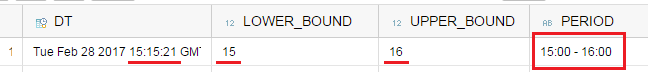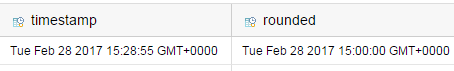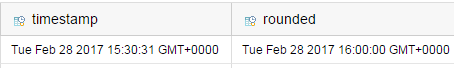SAP ABAP Programming and HANA Database Tutorials Development resources, articles, tutorials, code samples and tools and downloads for ASP.Net, SQL Server, R Script, Windows, Windows Phone, AWS, SAP HANA and ABAP, like SAP UI5, Screen Personas, etc.
 Home Articles News IT Jobs Tools Sample Chapters Trainers Blogs Forums Photos Files

Install SAP Free

# SQLScript Series_Round Function to Round DateTime in SAP HANA Database

Using SAP HANA SQLScript Series_Round() function, developers can calculate nearest datetime value in desired date or time interval using rounding for an input date time parameter. The date time interval can include any representation of time like day, hour, minutes, etc even in intervals like 5 minutes, 3 years, 30 seconds, etc.

SAP HANA SqlScript developers can refer to SAP Help Portal for detailed information and SQL code samples on Series_Round function

If you are developing SQL using Transact-SQL, Series_Round function might be different for you.
So to understand the Series_Round() series data function, it is better to see this SQLScript function in action.

Assume that you keep inserted datetime value on your database table for each row.
Besides inserted datetime value (as timestamp value), you want to keep the time of the insert moment as hourly period like "13:00 - 14:00" corresponding for an inserted date for example 13:19:26

Using Series_Round() SAP HANA SQL function with ROUND_FLOOR mode once for period lower time and ROUND_CEILING for interval upper time with an series interval value 1 hour, SQL programmer can easily get the desired period definition.

``` do begin  declare lv_ts TIMESTAMP := CURRENT_TIMESTAMP;  SELECT   :lv_ts dt,   HOUR( SERIES_ROUND(:lv_ts, 'INTERVAL 1 HOUR', ROUND_FLOOR) ) lower_bound,   HOUR( SERIES_ROUND(:lv_ts, 'INTERVAL 1 HOUR', ROUND_CEILING) ) upper_bound,   CAST( CAST( HOUR( SERIES_ROUND(:lv_ts, 'INTERVAL 1 HOUR', ROUND_FLOOR) ) as TIME) as VARCHAR(5))   || ' - ' ||   CAST( CAST( HOUR( SERIES_ROUND(:lv_ts, 'INTERVAL 1 HOUR', ROUND_CEILING) ) as TIME) as VARCHAR(5))   as period  FROM DUMMY; end; ```

For the above sample SQLScript code, the output after execution is as follows:Of course, if you are an experienced SQL developer you will not be impressed from the above SQLScript Series_Round function usage.

Assume that you want to round your datetime value to the nearest hour value. If the minutes part is equal to 30 or above, SQL developer should round to nearest upper value. Otherwise, if minutes section is less than 30, output should return the nearest lower value in hour part of the datetime value.

Here is the SQLScript code for this datetime rounding requirement.

``` SELECT  CURRENT_TIMESTAMP "timestamp",  SERIES_ROUND(CURRENT_TIMESTAMP, 'INTERVAL 1 HOUR') "rounded" FROM DUMMY; ```

I executed above SQLScript code on SAP HANA database at twice each at different times and got following results.
First CURRENT_TIMESTAMP value is rounded to lower bound value, because the minute part of time is less than 30 minutes.The second execution time is about 3 minutes later and the CURRENT_TIMESTAMP is rounded to upper bound limit.Let's work on another SQLScript code to see the series_round function with different interval options like minutes, hours, days and months.

I created a sample table in my SAP HANA database containing only a column with type datetime and populated the HANA table with sample data.

``` drop table "kodyaz dates"; create table "kodyaz dates" (  datetime datetime ); insert into "kodyaz dates" (datetime) values ('2017-02-26 18:27:16'); insert into "kodyaz dates" (datetime) values ('2017-02-12 11:17:36'); insert into "kodyaz dates" (datetime) values ('2017-02-18 10:45:30'); insert into "kodyaz dates" (datetime) values ('2017-02-19 23:50:06'); select  datetime,  series_round(datetime,'INTERVAL 15 MINUTE'),  series_round(datetime,'INTERVAL 6 HOUR'),  series_round(datetime,'INTERVAL 3 DAY'),  series_round(datetime,'INTERVAL 1 MONTH') from "kodyaz dates"; ```

Here is the output of the above SQL code execution.

If you make a mistake while defining the interval option in SERIES_ROUND function, the SAP HANA database engine will throw the below SQL exeption:

Error in reading value (type 16): dberror(getTimestamp): 293 - argument type mismatch: Increment_by units must be YEAR, MONTH, DAY, HOUR, MINUTE, or SECOND: at function series_round() (at pos 165)

I liked the datetime rounding function series_round because this SQLScript function enables me to avoid many datetime calculations using different functions and doing the exact job I want to accomplish in a single command.

SAP Tutorials

SAP Tutorial

SAP Forums

SAP Tools

SAP Transaction Codes Table

Meetup Sunumu 1